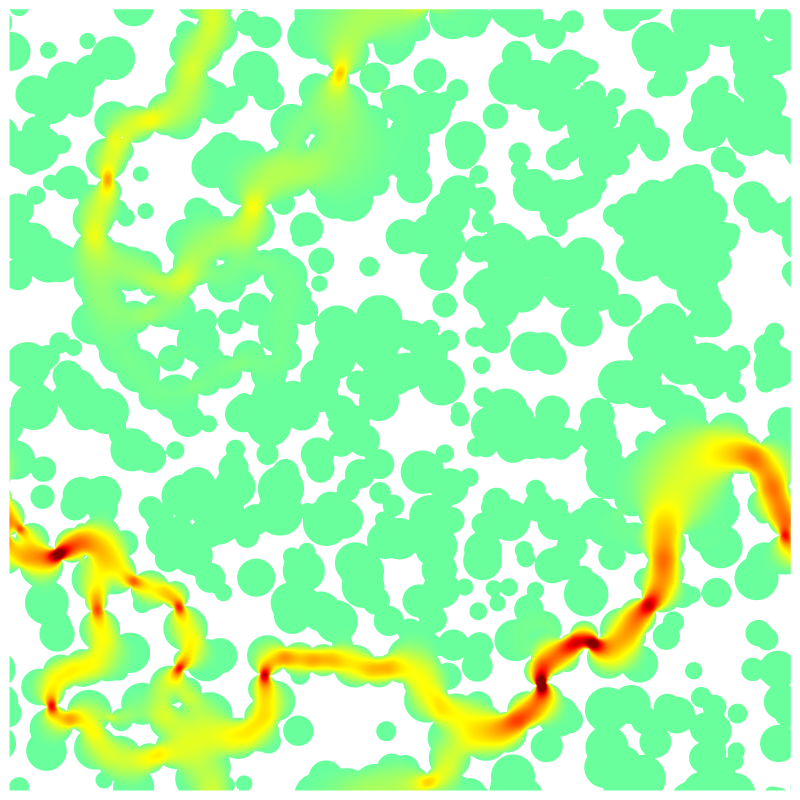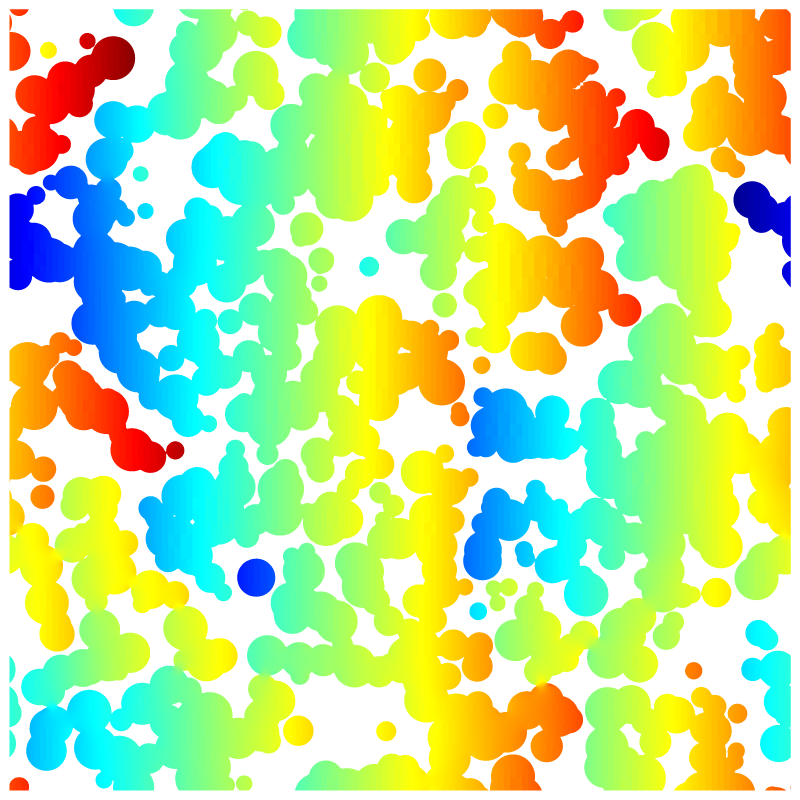# Stokes flow through a complex porous medium

The medium is periodic and described using embedded boundaries.

This tests mainly the robustness of the representation of embedded boundaries and the convergence of the viscous and Poisson solvers.

#include "embed.h"
#include "navier-stokes/centered.h"
#include "view.h"

We will vary the maximum level of refinement, starting from 5.

int maxlevel = 5;

The porous medium is defined by the union of a random collection of disks. The number of disks can be varied to vary the porosity.

void porous (scalar cs, face vector fs)
{
int ns = 800;
double xc[ns], yc[ns], R[ns];
srand (0);
for (int i = 0; i < ns; i++)
xc[i] = 0.5*noise(), yc[i] = 0.5*noise(), R[i] = 0.01 + 0.02*fabs(noise());

Once we have defined the random centers and radii, we can compute the levelset function \phi representing the embedded boundary.

  vertex scalar phi[];
foreach_vertex() {
phi[] = HUGE;

Since the medium is periodic, we need to take into account all the disk images using periodic symmetries.

    for (double xp = -L0; xp <= L0; xp += L0)
for (double yp = -L0; yp <= L0; yp += L0)
for (int i = 0; i < ns; i++)
phi[] = intersection (phi[], (sq(x + xp - xc[i]) +
sq(y + yp - yc[i]) - sq(R[i])));
phi[] = -phi[];
}
fractions (phi, cs, fs);
fractions_cleanup (cs, fs);
}

The domain is the periodic unit square centered on the origin.

int main()
{

Space and time are dimensionless. This is necessary to be able to use the ‘mu = fm’ trick.

  size (1. );

origin (-0.5, -0.5);
periodic (right);
periodic (top);

We turn off the advection term. The choice of the maximum timestep and of the tolerance on the Poisson and viscous solves is not trivial. This was adjusted by trial and error to minimize (possibly) splitting errors and optimize convergence speed.

  stokes = true;
DT = 2e-5 ;
#if 1
TOLERANCE = HUGE;
NITERMIN = 2;
#else
TOLERANCE = 1e-3;
NITERMIN = 2;
#endif
N = 1 << maxlevel;

run();
}

scalar un[];

event init (t = 0) {

We define the porous embedded geometry.

  porous (cs, fs);

The gravity vector is aligned with the channel and viscosity is unity.

  const face vector g[] = {1.,0.};
a = g;
mu = fm;

The boundary condition is zero velocity on the embedded boundary.

  u.n[embed] = dirichlet(0);
u.t[embed] = dirichlet(0);

We initialize the reference velocity.

  foreach()
un[] = u.x[];
}

We check for a stationary solution.

event logfile (i++; i <= 500)
{
double avg = normf(u.x).avg, du = change (u.x, un)/(avg + SEPS);
fprintf (stderr, "%d %d %d %d %d %d %d %d %.3g %.3g %.3g %.3g %.3g\n",
maxlevel, i,
mgp.i, mgp.nrelax, mgp.minlevel,
mgu.i, mgu.nrelax, mgu.minlevel,
du, mgp.resa*dt, mgu.resa, statsf(u.x).sum, normf(p).max);

If the relative change of the velocity is small enough we stop this simulation.

  if (i > 1 && (avg < 1e-9 || du < 1e-2)) {

We are interested in the permeability k of the medium, which is defined by \displaystyle U = \frac{k}{\mu}\nabla p = \frac{k}{\mu}\rho g with U the average fluid velocity.

    stats s = statsf (u.x);
printf ("%d %g\n", maxlevel, s.sum/s.volume);

We output fields and dump the simulation.

    scalar nu[];
foreach()
nu[] = sqrt (sq(u.x[]) + sq(u.y[]));

view (fov = 19.3677);

draw_vof ("cs", "fs", filled = -1, fc = {1,1,1});
squares ("nu", linear = true, spread = 8);
char name;
sprintf (name, "nu-%d.png", maxlevel);
save (name);

draw_vof ("cs", "fs", filled = -1, fc = {1,1,1});
squares ("p", linear = false, spread = -1);
sprintf (name, "p-%d.png", maxlevel);
save (name);

draw_vof ("cs", "fs", filled = -1, fc = {1,1,1});
squares ("level");
sprintf (name, "level-%d.png", maxlevel);
save (name);

sprintf (name, "dump-%d", maxlevel);
dump (name);

We stop at level 10.

    if (maxlevel == 10)
return 1; /* stop */

We refine the converged solution to get the initial guess for the finer level. We also reset the embedded fractions to avoid interpolation errors on the geometry.

    maxlevel++;
#if 0
refine (level < maxlevel && cs[] > 0. && cs[] < 1.);
#else
#endif
porous (cs, fs);
}
}Norm of the velocity field.Pressure field.Adapted mesh, 10 levels of refinement.

set xlabel 'Level'
set grid
set ytics format '%.1e'
set logscale y
plot 'out' w lp t ''Permeability as a function of resolution (script)

set xlabel 'Iterations'
set logscale y
set ytics format '%.0e'
set yrange [1e-10:]
plot '../porous.ref' u 2:9 w l t '', '' u 2:10 w l t '', \
'' u 2:11 w l t '', '' u 2:12 w l t '', '' u 2:13 w l t '', \
'log' u 2:9 w p t 'du', '' u 2:10 w p t 'resp', \
'' u 2:11 w p t 'resu', '' u 2:12 w p t 'u.x.sum', '' u 2:13 w p t 'p.max'Convergence history (script)